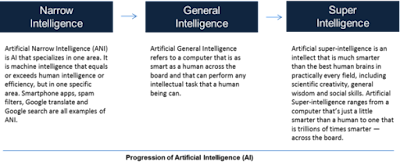Showing posts with label Schwartz Information Criteria. Show all posts
Showing posts with label Schwartz Information Criteria. Show all posts

### AI Glossary - AIC Or Akaike Information Criteria.

Akaike Information Criteria or the AIC is a data-driven metric for evaluating several models for the same set of data.

It was calculated by taking into account the loss of accuracy in a model when data-based estimations of the model's parameters were substituted for the true values.

A constant term specified by the real model, -2 times the probability for the data given the model, and a constant multiple (2) of the number of parameters in the model make up the equation for this loss.

The first phrase, involving the unknown true model, may be omitted since it enters as a constant (for a given set of data), leaving two known terms to be assessed.

AIC is the sum of a (negative) measure of the model's mistakes plus a positive penalty for the number of parameters in the model, expressed algebraically.

Increasing the model's complexity will increase the AIC only if the fit (as judged by the data's log-likelihood) improves more than the cost of the new parameters.

A group of competing models may be compared by calculating their AIC values and selecting the model with the lowest AIC value, implying that this model is the most similar to the real model.

Unlike traditional statistical methods, this enables for the comparison of models with no shared parameters.

### Related Terms:

Kullback-Liebler information measure, Schwartz Information Criteria

~ Jai Krishna Ponnappan